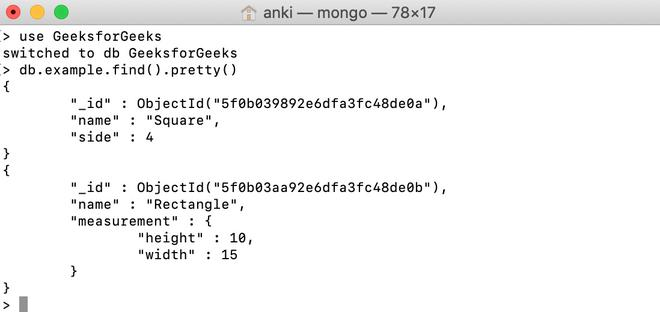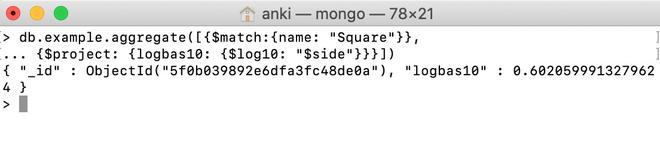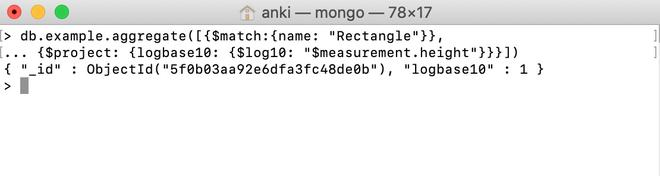Skip to content
Related Articles
MongoDB \$log10 Operator
• Last Updated : 28 Jul, 2020

MongoDB provides different types of arithmetic expression operators that are used in the aggregation pipeline stages and \$log10 operator is one of them. This operator is used to find the log base 10 of the specified number and returns the result as a double.

Syntax:

```{ \$log10: <number> }
```

Here, the number is a valid expression until it resolves to a non-negative number.

• If the entered value is null, then this operator will return null.
• If the entered value is NaN, then this operator will return NaN.
• If the entered value is a missing field, then this operator will return null.

Examples:

In the following examples, we are working with:

Database: GeeksforGeeks

Collection: example

Document: two documents that contain the details of the shapes in the form of field-value pairs.Using \$log10 operator:

In this example, we are going to find the log base 10 of the value of the side field in the square document.

```db.example.aggregate([{\$match:{name: "Square"}},
... {\$project: {logbas10: {\$log10: "\$side"}}}])

```Using \$log10 operator in the embedded document:

In this example, we are going to find the log base 10 of the value of the measurement.height field in the rectangle document.

```db.example.aggregate([{\$match:{name: "Rectangle"}},
... {\$project: {logbase10: {\$log10: "\$measurement.height"}}}])

```My Personal Notes arrow_drop_up